# Machine Learning Questions and Answers (Questions 21 to 30)

Welcome back! So we’ll continue with Questions 21 to 30 of our Machine Learning Q&A.

You can find Question 1 to 20 below

##### 21. What is Regularization?

Regularization is a technique used to control overfitting and it involves adding some penalty term to the model so that model’s coefficient does not reach very large values. One such penalty term is the sum of squares of all the coefficients w.

So if we have the sum of squares error to be: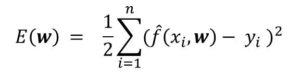then adjusted error function with  the penalty term is given by: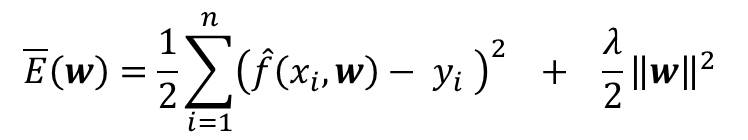where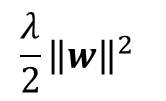is the regularization term or penalty term

an the λ prevents the penalty term from being too big or too small compared to the error term

##### 22. Differentiate between Regularization and Generalization

While regularization is a technique used to control overfitting adding a term that limits the variance of the model, generalization is a technique used to control underfitting.

Hence generalization is used to describe the behavior of the model that allows it be able make good predictions with new data.

##### 23. How can you minimize misclassification in a classification model

First, let’s remember that misclassification occur when an observation is assigned to the wrong class.

For a set of observations x, and classes Ck,  we find joint probability of assigning the the observation x to Classes Ck. This is given by p(x, Ck).

From Bayes’ Theorem, we can find the joint probability using conditional probability, thus:

p(x, Ck) = p(Ck | x) p(x)

Therefore, misclassification can be minimized if x is assigned to the class that has the largest posterior probability p(Ck | x)

##### 24. What is expected loss in classification? How can you minimize it?

First, expected loss is the lost incurred as a result of making a given decision. Similarly, a loss function or cost function is a measure of this loss. (opposite of this is the utility function).  A loss occurs if x is assigned to class Cj whereas j may not be equal to k).

We can then denote the loss incurred as Lkj. To minimize the expected loss, therefore, we need to minimize the function which is given by: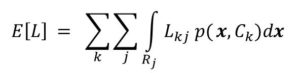I recommend you watch the video lesson for clearer understanding.

##### 25. What is a decision tree. What is it used for?

A decision tree is a tree-like structure that is obtained by continually partitioning the predictor variables (feature set) until a decision is reached. I can be used in supervised learning to perform both classification and regression.

In a decision tree, the selection process is a sequence of binary selections at each step that match the traversal of tree structure.

##### 26. Explain Logistic Regression

Logistic regression which is also called binary regression is a type of regression used to binary events. It is actually a classification model where the response variables are simple one of two classes.

##### 27. State the logistic regression Model

The logistic regression function models the probability of x p(X) as a function that outputs either values between 0 and 1 for all the values of X. This is given by: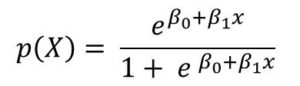Also watch the video

##### 28. What are odds ratio in Logistic Regression?

Odds is another way is representing probabilities which can be derived from rearranging the logistic function. Unlike probabilities, odds take values from 0 to ∞.  The value of odds close to 0 indicates low probability while value of odds close to ∞  represents high probability.

Odds is given by:##### 29. How is neural network related to classification and regression

Just like models for classification and regression, neural networks can also be used to build models that can be used to make inference or prediction. Similar to basis functions in classification, neural networks are based on activation function whose parameters needs to be determined and optimized.

##### 30. How is Bayes’ Theorem related to logistic regression

Logistic regression is a probabilistic model that is based on Bayes’ Theorem to determine the class with the largest probability for given observation.#### kindsonthegenius

Kindson Munonye is currently completing his doctoral program in Software Engineering in Budapest University of Technology and Economics

View all posts by kindsonthegenius →